﻿ 管道中滞留气团排放过程的二维数值模拟研究

管道中滞留气团排放过程的二维数值模拟研究A 2-D Numerical Simulation Study on the Process of Air Mass Emission in Pipeline

Abstract: Under severe rainstorm conditions, transient flow of trapped air masses often occurs in urban storm water drainage pipelines. In this paper, VOF (Volume of Fluid Model) model and k-ε turbu-lence model are used to simulate and calculate the dynamic process of the movement and emission of the stranded gas mass under the action of pressure flow. The simulation results are compared with the experimental results. The results show that the motion process and transient pressure variation of the trapped air masses simulated by the VOF model agree well with the experimental results. At the same time, the two simplified methods, such as “equal section ratio” and “equal diameter ratio”, are compared and analyzed in two-dimensional simulation. The comparison results show that the pressure result calculated by the “equal section ratio” method is closer to the experimental value.

1. 引言

2. 数学模型

2.1. 控制方程

$\frac{\partial \rho }{\partial t}+\nabla \cdot \left(\rho v\right)=0$ (1)

$\frac{\partial \rho {u}_{i}}{\partial t}+\frac{\partial \rho {u}_{i}{u}_{j}}{\partial {x}_{j}}=\frac{\partial p}{\partial {x}_{i}}+\frac{\partial {\tau }_{ij}}{\partial {x}_{j}}+\rho {g}_{i}$ (2)

$\frac{\partial {\alpha }_{w}}{\partial t}+v\nabla \cdot {\alpha }_{w}=0$ (3)

$\frac{\partial \rho E}{\partial t}+\frac{\partial }{\partial {x}_{j}}\left[{u}_{i}\left(\rho E+p\right)\right]=\frac{\partial }{\partial {x}_{i}}\left({k}_{eff}\frac{\partial T}{\partial {x}_{i}}\right)$ (4)

$\frac{\partial }{\partial t}\left({\alpha }_{w}{\rho }_{w}\right)+\nabla \cdot \left({\alpha }_{w}{\rho }_{w}U\right)=0$ (5)

${\alpha }_{w}+{\alpha }_{a}=1$ (6)

$\rho =\left({\alpha }_{w}{\rho }_{w}\right)+\left(1-{\alpha }_{w}\right){\rho }_{a}$ (7)

$\left\{\begin{array}{l}\frac{\partial \rho k}{\partial t}+\frac{\partial \rho k{u}_{i}}{\partial {x}_{i}}=\frac{\partial }{\partial {x}_{j}}\left[\left(\mu +\frac{{\mu }_{t}}{{\sigma }_{k}}\right)\frac{\partial k}{\partial {x}_{j}}\right]+{G}_{k}+{G}_{b}+\rho \epsilon -{Y}_{M}\\ \frac{\partial \rho \epsilon }{\partial t}+\frac{\partial \rho \epsilon {u}_{i}}{\partial {x}_{i}}=\frac{\partial }{\partial {x}_{j}}\left[\left(\mu +\frac{{\mu }_{t}}{{\sigma }_{\epsilon }}\right)\frac{\partial \epsilon }{\partial {x}_{j}}\right]+{C}_{1\epsilon }\frac{\epsilon }{k}\left({G}_{k}+{C}_{3\epsilon }{G}_{b}\right)-{C}_{2\epsilon }\frac{\rho {\epsilon }^{2}}{k}\end{array}$ (8)

${\mu }_{t}=\rho {C}_{\mu }\frac{{k}^{2}}{\epsilon }$ (9)

2.2. 求解方法

2.3. 边界条件

1) 进口边界条件设置。进水口断面为压力进口，在实验过程中为系统提供稳定的水压。

2) 出口边界条件设置。出口边界为压力出口，相对压力为零。

3) 管道壁条件设置。壁面为光滑壁面，粗糙度为0。

3. 二维建模

3.1. 算例介绍

3.2. 二维建模的简化方法

$\frac{a}{b}=\frac{d}{D}$ (10)

$\frac{ax}{bx}=\frac{\text{π}{d}^{2}/4}{\text{π}{D}^{2}/4}⇒\frac{a}{b}=\frac{{d}^{2}}{{D}^{2}}$ (11)

3.3. 二维模型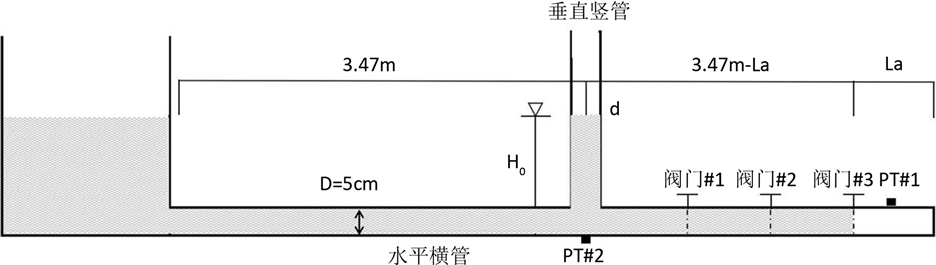Figure 1. Physical experimental apparatus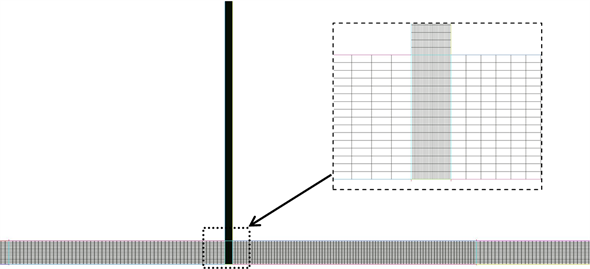Figure 2. Model gridTable 1. Four calculation model parameters

4. 计算结果及数据分析

4.1. 滞留气团排放过程的动态分析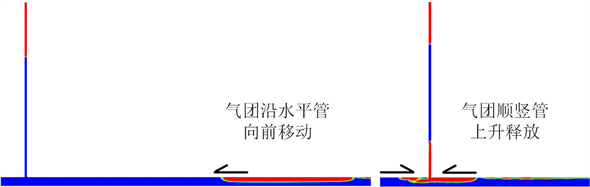Figure 3. Working condition (Bd) simplified gas-liquid phase cloud diagram with constant diameter ratio

4.2. 瞬态压力分析

4.3. 两种简化方法对比及分析

$\eta ={d}^{2}/{D}^{2}$ (12)

$\mu =\frac{d\ast h}{D\ast h}=d/D$ (13)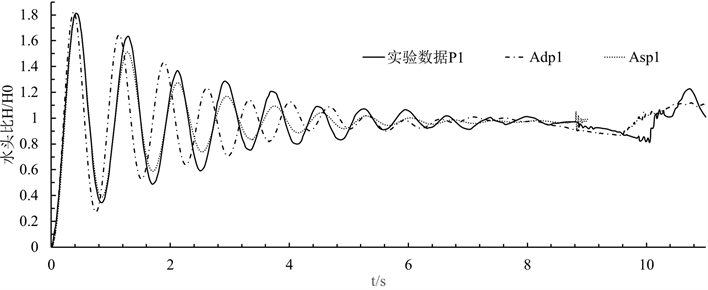Figure 4. Test data of pressure sensor #1 in working condition A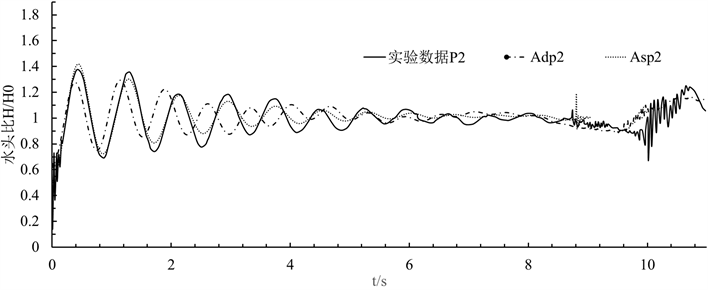Figure 5. Test data of pressure sensor #2 in working condition A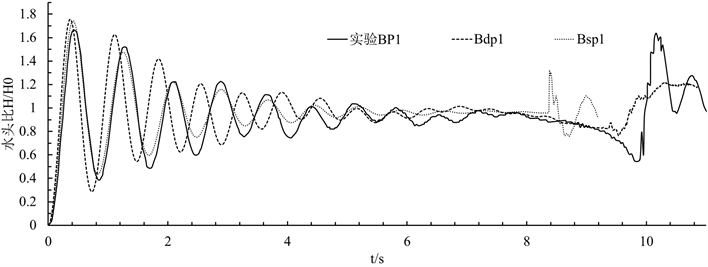Figure 6. Test data of pressure sensor #1 in working condition B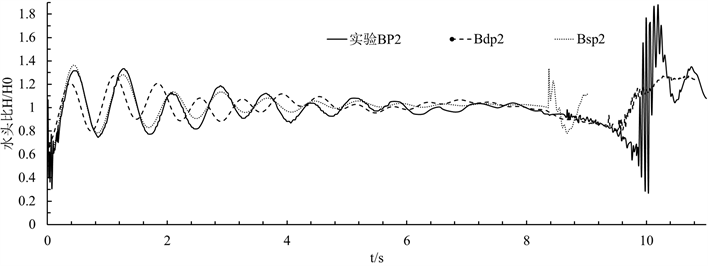Figure 7. Test data of pressure sensor #2 in working condition A

5. 结论

1) 在二维建模时，“等直径比例”与“等截面比例”两种简化方法的对比表明：可以按实际管道的截面比例建立模型，该模型可以有效的控制模型中横竖管的流通能力，令模拟计算结果更接近实际值。按现实管道的直径比例建立的模型，会放大细管截面积，增大细管流通能力，从而影响计算结果。

2) VOF模型可用于模拟滞留气团的运动过程、瞬态压力变化结果等气水动态行为和瞬变压力，模拟结果完全满足研究需要，并且具有一定的参考价值。

 Huang, B., Wu, S., Zhu, D.Z., et al. (2018) Experimental Study of Geysers through a Vent Pipe Connected to Flowing Sewers. Water Science & Technology, 2018, Article ID: t2018085.
https://doi.org/10.2166/wst.2018.085

 Cong, J., Chan, S.N. and Lee, J.H.W. (2017) Geyser Formation by Release of Entrapped Air from Horizontal Pipe into Vertical Shaft. Journal of Hydraulic Engi-neering, 143.
https://doi.org/10.1061/(ASCE)HY.1943-7900.0001332

 Muller, K.Z., Wang, J. and Vasconcelos, J.G. (2017) Water Displacement in Shafts and Geysering Created by Uncontrolled Air Pocket Releases. Journal of Hydraulic Engineering, 143.

 刘德有, 周领, 索丽生, 等. 水流冲击管道内滞留气团现象的VOF模型仿真分析[J]. 计算力学学报, 2009, 26(3): 390-394.

 马佳杰, 周领, 刘德有, 等. 管内水流冲击滞留气团的CFD模拟方法验证[J]. 水电能源科学, 2016(12): 110-113.

 Zhou, L.D., Liu, D., Karney, B., et al. (2013) Phenomenon of White Mist in Pipelines Rapidly Filling with Water with Entrapped Air Pockets. Journal of Hydraulic Engineering, 139, 1041-1051.
https://doi.org/10.1061/(ASCE)HY.1943-7900.0000765

 Zhou, L.D., Liu, D. and Karney, B. (2013) Investigation of Hy-draulic Transients of Two Entrapped Air Pockets in a Water Pipeline. Journal of Hydraulic Engineering, 139, 949-959.
https://doi.org/10.1061/(ASCE)HY.1943-7900.0000750

 郭艳惠, 刘德有, 周领. 关阀过程中管内瞬变流一二维数值模拟对比[J]. 人民黄河, 2013, 35(9): 122-124.

 Zhou, L., Liu, D. and Ou, C. (2011) Simulation of Flow Transients in a Water Filling Pipe Containing Entrapped Air Pocket with VOF Model. Engineering Applications of Computational Fluid Mechanics, 5, 127-140.
https://doi.org/10.1080/19942060.2011.11015357

 李东风, 潘杰, 陈海雄, 等. 河流连通工程防洪排涝影响二维数值模拟[J]. 人民黄河, 2016, 38(11): 31-33.

 张根广, 高改玉, 田艳, 等. 横丹水电站下游冲刷坑二维数值模拟[J]. 应用基础与工程科学学报, 2017(6): 1139-1146.

Top